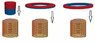# Different magnets with a coil, what are the currents?

• Holchr
If not, Lenz's Law states that the EMF (force exerted on the magnet by the field of the induced current) is inversely proportional to the current through the coil, and voltage is proportional to the number of turns in the coil.Thanks.

#### Holchr

Hi.
Can someone please explain/tell me what would be the resulting currents trough the coil in every of the three depicted scenarios in the attached picture, I'm confused here. Would the first and the second drawing produce the same result? Is there any current flow in the third drawing.
Thanks.

#### Attachments

•magnetsandcoils.jpg
28.8 KB · Views: 427
Holchr said:
Hi.
Can someone please explain/tell me what would be the resulting currents trough the coil in every of the three depicted scenarios in the attached picture, I'm confused here. Would the first and the second drawing produce the same result? Is there any current flow in the third drawing.
Thanks.

The first and second will produce current in opposite direction(since change in magnetic flux is opposite in direction, due to, magnetic fields through the coil are in opposite direction), there is NO current in the third picture because of NO flux through coil(assuming the magnet is big enough to not have edge effects).

Thanks a lot universal. I got just one more question so I'd really appreciate help.

When a magnet passes trough a coil, there are three points of interest to me. The VOLTAGE, the CURRENT, and the EMF (lenz effect, or the force exerted on the magnet by the field of the induced current) (maybe some of this is wrong but bear with me).

So I wanted to know to which of the parameters of the system are VOLTAGE, CURRENT and the EMF proportional, and to which inversely proportional, or is not affected by the parameter.

1. The number of turns in a coil
2. The diameter of the wire in a coil
3. The magnetic flux density of the permanent magnet
4. The velocity of the magnet trough the coil.

Thanks.

Holchr said:
Thanks a lot universal. I got just one more question so I'd really appreciate help.

When a magnet passes trough a coil, there are three points of interest to me. The VOLTAGE, the CURRENT, and the EMF (lenz effect, or the force exerted on the magnet by the field of the induced current) (maybe some of this is wrong but bear with me).

So I wanted to know to which of the parameters of the system are VOLTAGE, CURRENT and the EMF proportional, and to which inversely proportional, or is not affected by the parameter.

1. The number of turns in a coil
2. The diameter of the wire in a coil
3. The magnetic flux density of the permanent magnet
4. The velocity of the magnet trough the coil.

Thanks.

The answers to most of your questions are covered by Lenz's Law:

http://en.wikipedia.org/wiki/Lenz's_law

Are you familiar with it yet?